•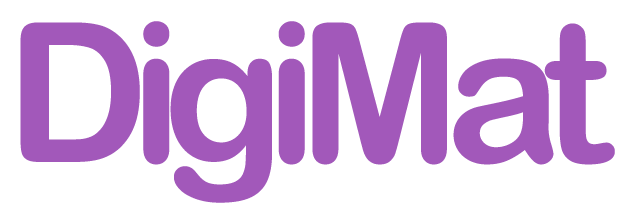• Learn mathematical programming!
• Makes coding a natural part of math
• For all levels
• Creative and high impact activities

Contact: Johan Jansson jjan@kth.se

DigiMat is part of the Digital Math research environment: http://digitalmath.tech

•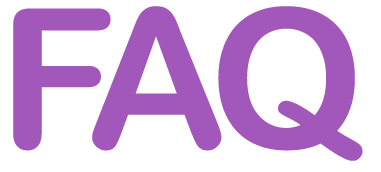## What is Mathematical Programming?

Mathematical programming unifies math and coding. It’s based on scientifically grounded physics simulations, which means computing and predicting scenarios with a computer. With DigiMat you’ll learn to create your own virtual worlds.

## Who is DigiMat for?

DigiMat is for everyone, no matter age or level. Kids, parents, teachers and university students and professionals. Anyone can learn to use the simple algorithms of DigiMat.

## Why learn Mathematical Programming?

Mathematical Programming is crucial for industry and our digital society. With mathematical programming you can do much more powerful, interesting and useful things than with regular coding.

## What didactic method does DigiMat use?

DigiMat focuses on motivation, creativity and learning by doing. Motivation is key to store information in the long term memory. By focusing on the amazing things you can achieve you'll find motivation to learn more and reach higher. With mathematical programming you get a better understanding of the algorithms rather than learning special cases by heart, which is what traditional math education often focus on. You learn that the basics of math and coding are the same, and by that you understand the basic principles of both mathematics and programming.

## Which are the DigiMat learning goals?

1. Number representation [Basic] in first binary form making representation and arithmetic algorithms easy to understand.
2. Arithmetic algorithms [Basic] constructed by repetition of the basic operation of +1 according the basic prototype of all computer programs of DigiMat in the form n = n + 1
3. Time-stepping [Basic-Pro] automatically solving all (ordinary) mathematical models in the form x = x + v*dt
4. Text programing [Basic-Pro] enabling the students to understand, modify and extend the algorithms and computer realizations themselves.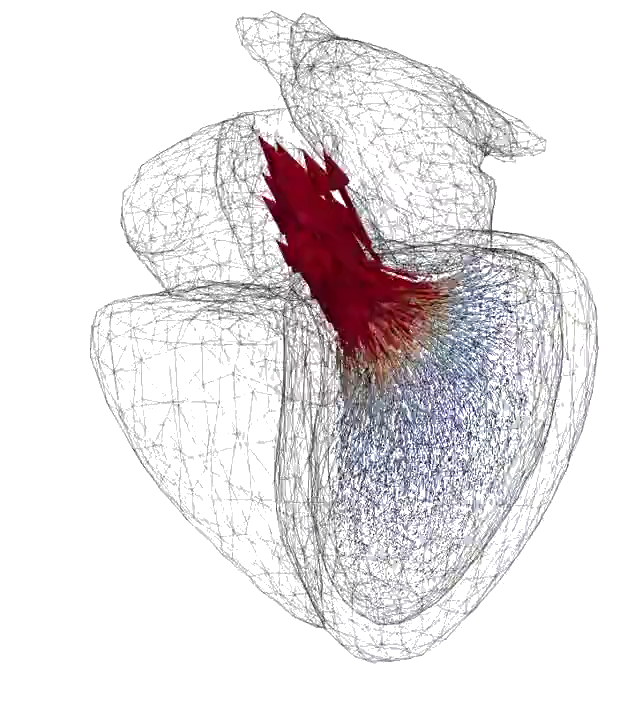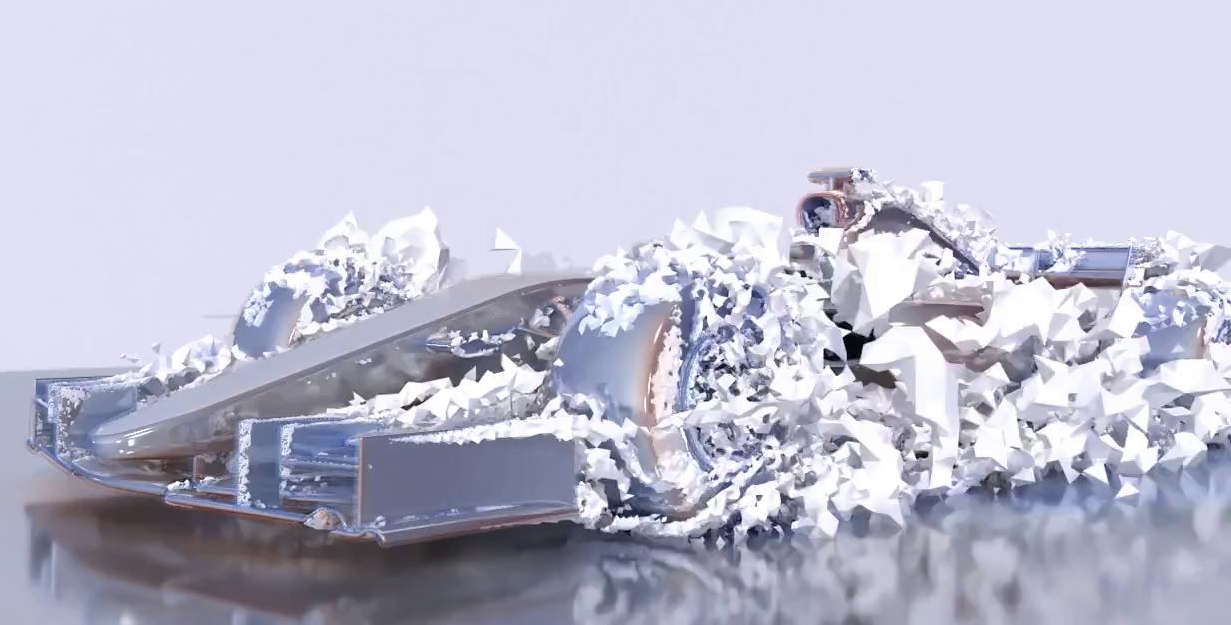•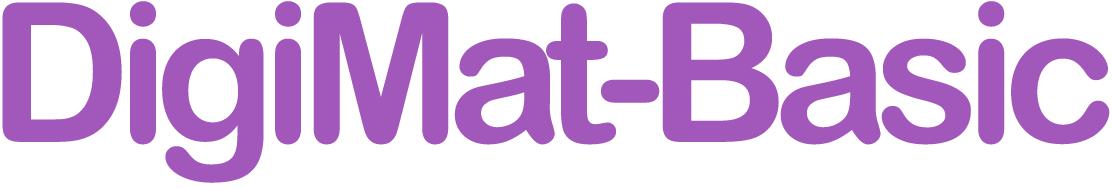Enter Ada's World! Our pedagogical app is inspired by Ada Lovelace (1815-1852), mathematician and one of the first programmers in history. Here you learn the binary addition algorithm and time-stepping for simulation, by organizing a party. You'll also meet and learn about Ada’s friends, all based on leading personas through the history of math and programming.

•DigiMat Mid is for you who are a little more experienced and feel comfortable with the algorithms (arithmetic and basic time stepping) and text programming in DigiMat Basic.

In DigiMat Mid you navigate a world with activities, and build your own virtual worlds around them, for example focusing on:

1. Games
2. Dance - motion, music
3. Design - vehicles, buildings, etc.
4. Medicine
5. Society

Here are examples of activities: Showcase: Trigonometry with time stepping
DigiMat Learning activity

1. Binary Representation of Natural Numbers
DigiMat Learning Activity

2. Motion-Change: x = x + v*dt or dx = v*dt
DigiMat Learning Activity

DigiMat Learning Activity

DigiMat Learning Activity

5. Binary Abacus 1
DigiMat Learning Activity

6. Binary Abacus 2
DigiMat Learning Activity

DigiMat Learning Activity

8. Binary Multiplication
DigiMat Learning Activity 1
DigiMat Learning Activity 2

9. Binary Subtraction
DigiMat Learning Activity 1
DigiMat Learning Activity 2

10. Binary Subtraction
DigiMat Learning Activity 1
DigiMat Learning Activity 2

11. Natural Numbers: Base 3
DigiMat Learning Activity

12. Natural Numbers: Any Base
DigiMat Learning Activity

13. Binary Division
DigiMat Learning Activity

14. Pocket Calculator
DigiMat Learning Activity

15. Primes
DigiMat Learning Activity

16. Fibonacci Numbers
DigiMat Learning Activity

17. Screen Geometry
DigiMat Learning Activity

18. Motion on Screen
DigiMat Learning Activity

19. Draw Line
DigiMat Learning Activity

20. Newtonian Mechanics: Throw Birds Basic
DigiMat Learning Activity

21. Newtonian Mechanics
DigiMat Learning Activity

22. Polynomials
DigiMat Learning Activity

23. Exponential Function exp(t)
DigiMat Learning Activity

24. Natural Logarithm log(t)
DigiMat Learning Activity

25. Natural Logarithm log(t)
DigiMat Learning Activity

26. Harmonic Series
DigiMat Learning Activity

27. Draw Circle
DigiMat Learning Activity

28. Solving f(x)=0 by Bisection
DigiMat Learning Activity

29. Solving f(x)=0 by Time Stepping x = x + f(x)*dt
DigiMat Learning Activity

30. Solving f(x)=0 by Time Stepping x = x + f(x)*dt
DigiMat Learning Activity

31. Solving x=g(x) by Fixed Point Iteration
DigiMat Learning Activity

32. Calculus as dx = f(t)dt as x = integral f(t)dt
DigiMat Learning Activity

33. Time stepping: Smart, Dumb and Midpoint Euler
DigiMat Learning Activity

34. Integral: Midpoint Euler vs Forward/Endpoint Euler
DigiMat Learning Activity

35. Compute Area of Circular Disk. Compute Pi.
DigiMat Learning Activity

36. Level Curves in 2D
DigiMat Learning Activity

#### DigiMat Basic Books and More Material

There is an extensive list of books supporting DigiMat.

Books gives the foundation of the path and lead into a wider world as computational mathematics.

DigiMat is an expansion of the BodyandSoul program, which contains supporting material, some of the software material may not be supported anymore.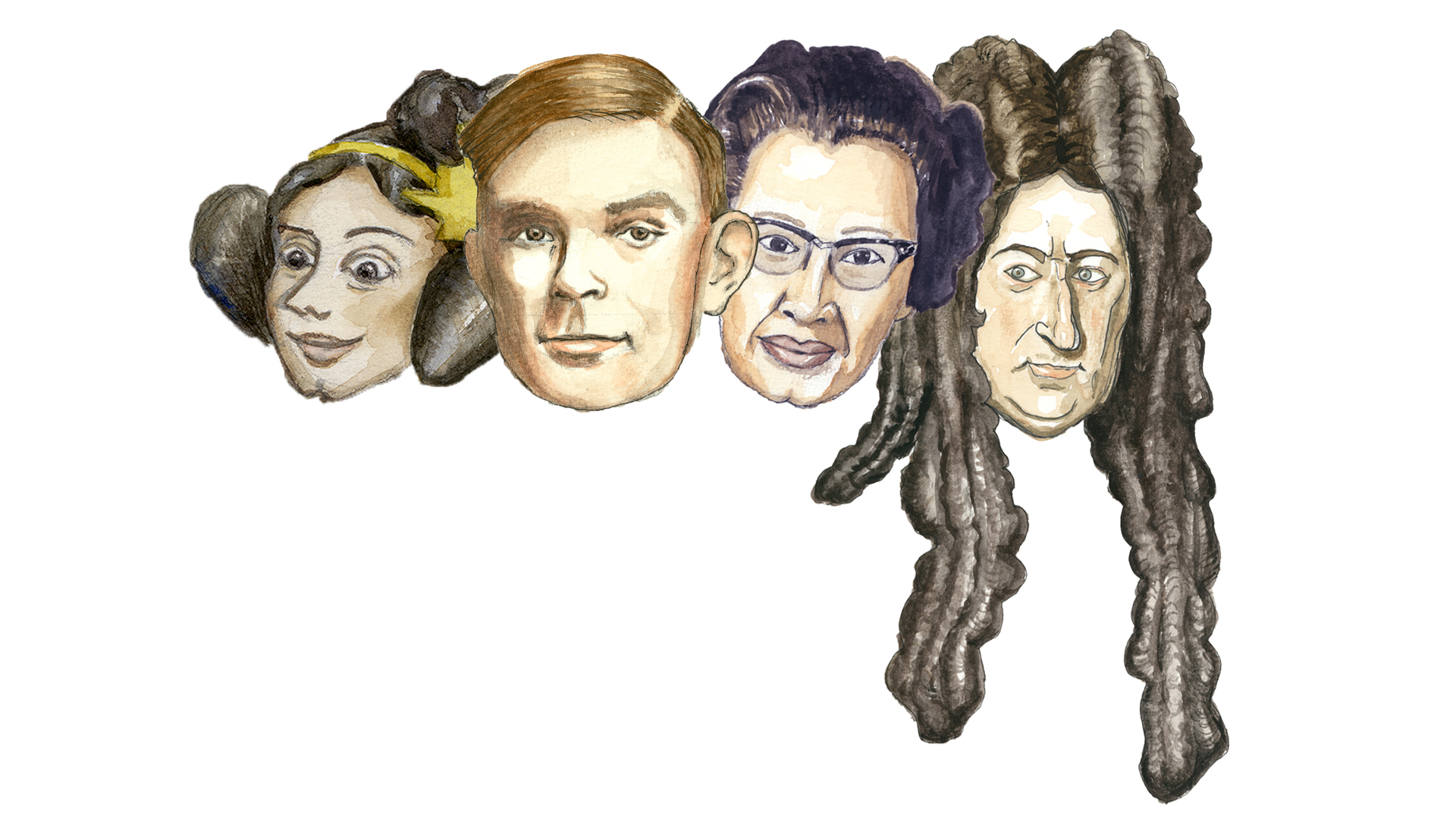•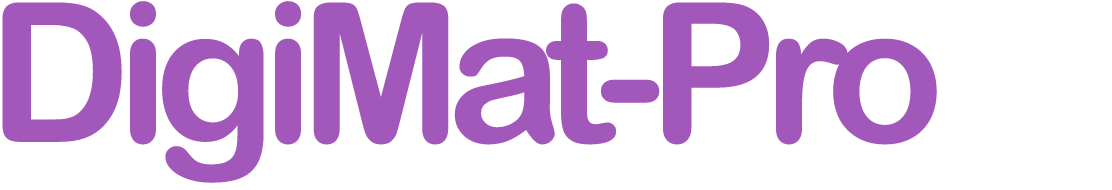• This is the advanced DigiMat course for university level, professionals and experienced programmers.
• Get started here and now! You'll find the entire course with videos and interactive learning activities in the modules below.
• To receive a course certificate for a fee of 100 USD, e-mail course leader Johan Jansson, jjan@kth.se. Or pay directly through Stripe with this link: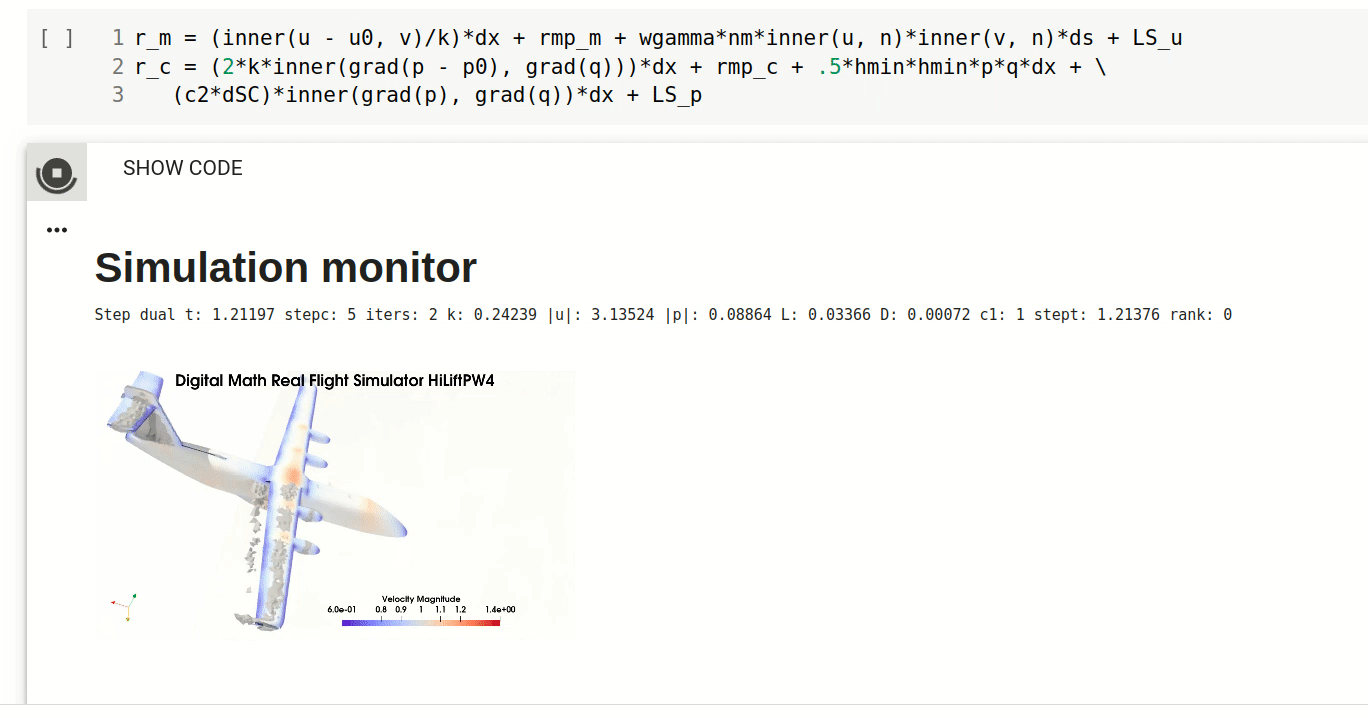Example of DigiMat Pro environment

1. Understand and try how Digital Math underlies science and industry, and allows prediction of the mathematical models governing reality.

2. Galerkin's method part 1. Understand the finite element method in a familiar linear algebra setting. Learn how the finite elelement method is the best possible method for several classes of problems.

3. Adaptive error control. Learn how to optimally apply the finite element discretization with adaptivity for fast and cheap computation.

4. Get an overview of high performance and advanced predictive aerodynamics applications and learn about performance in an adaptive FEM and HPC setting.

5. Understand the mechanism of flight, aerodynamics and turbulence and how to efficiently predict flight simulation with Real Flight Simulation in Digital Math.

#### You can also enter DigiMat Pro in the CMU-OLI system in collaboration with Carnegie-Mellon Open Learning Initiative.

•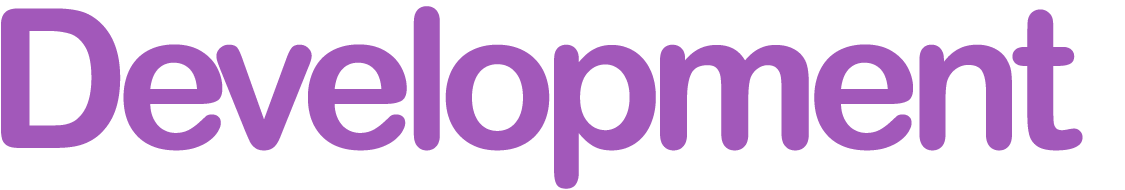Here you'll find small modules or prototypes which can be edited and structured into cohesive and pedagogical learning activities.

Start contributing to DigiMatPedia! We suggest starting from existing prototypes in p5.js and/or FEniCS and adapting to the DigiMat framework. Email Johan Jansson (jjan@kth.se) to include your contribution on this page.

#### Billiards

##### TODO
1. Add "visual cues" e.g. hands/fingers indicating to click on the white ball, indicating to move values into the "variable box", etc.

#### Rocket

##### Level 1

Reference:
https://www.ncbi.nlm.nih.gov/pmc/articles/PMC7321055/

#### Machine Learning with P5.js

Reference:
https://thecodingtrain.com/CodingChallenges/100.1-neuroevolution-flappy-bird.html
##### Level 1 - Flappy Bird Game
• Upplev Adas Värld! Vår pedagogiska app är inspirerad av Ada Lovelace (1815-1852), matematiker och en av de första programmerarna i historien. Här lär du dig den binära additionsalgoritmen och tidsstegning för simulering, genom att ordna en fest. Du kommer också träffa och lära dig om Adas vänner, baserade på framstående personer från matematikens och programmeringens historia.

För att komma igång, se workshop-aktiviteterna nedan!

### Workshopens läraktiviteter

1. Se på trailervideon på DigiMats huvudsida!
2. Se på introvideon till höger
3. Skapa en dans! (Ada's World level 1) I Ada's World: Gör en danssimulering, och skapa en egen dans!

4. Simulering - Angry Birds! (Ada's World level 1) I Ada's World: Gör tidssteningsalgoritmen i "kastsimuleringen".

5. Tidsstegning av vibration i en gitarrsträng (Ada's World level 2)

Lek med den digitala matematikkoden för tidsstegning. Välj olika frekvenser/tonhöjder i listan, lägg till och ta bort värden, vad händer?

Avancerat: ändra "dämpningen" i gitarrsträngen genom att lägga till termen " - y*k" till "y = y +"... raden. Vad händer med ljudet när du ökar eller minskar dämpningen?

### Feedback för Workshopen

Vänligen ge feedback i formuläret nedan!
2. Var det något som var svårt att förstå?
3. Vad skulle du vilja göra mer i DigiMat, om du fick välja helt själv?

### Workshopens lärandemål

Workshop 01 för DigiMat innehåller de följande fundamentala lärandemålen, som är grunden för hela DigiMat

1. Talrepresentation [Basic] först i binär form som gör representation och aritmetiska algoritmer enkla att förstå.
2. Artimetiska algoritmer [Basic] konstruerade genom repetition av den fundamentala operationen +1 enligt den grundläggande prototypen för alla datorprogram i DigiMat i formen n = n + 1
3. Tidsstegning [Basic-Pro] automatiskt löser alla ordinära matematiska modeller i formen x = x + v*dt
4. Textprogrammering [Basic-Pro] gör det möjligt för elever att förstå, modifiera och utöka algoritmerna och datorrealiseringarna själva.

Ada's World Level 1: Danssimulering med textprogrammering - Tutorial!

Ada's World Level 2: Vibrerande gitarrsträng - Tutorial!

Ada's World Level 1: Tidsstegning nivå 1 !

### Extramaterial

Ada's World Level 2: Textprogrammering med tidsstegning nivå 1 !

Ada's World Level 2: Textprogrammering med tidsstegning nivå 2 !

Ada's World Level 2: Textprogrammering med tidsstegning nivå 3 !

Ada's World Level 2: Textprogrammering med tidsstegning nivå 4 !

• Upplev Adas Värld! Vår pedagogiska app är inspirerad av Ada Lovelace (1815-1852), matematiker och en av de första programmerarna i historien. Här lär du dig den binära additionsalgoritmen och tidsstegning för simulering, genom att ordna en fest. Du kommer också träffa och lära dig om Adas vänner, baserade på framstående personer från matematikens och programmeringens historia. T.ex. Alan Turing som var med och skapade den moderna datorn.

För att komma igång, se workshop-aktiviteterna nedan.

### Workshopens läraktiviteter

1. Se på trailervideon på DigiMats huvudsida!
2. Se på introvideon till höger
3. Skapa en dans! (Ada's World level 1) I Ada's World: Gör en danssimulering, och skapa en egen dans.

4. Simulering - Angry Birds (Ada's World level 1) I Ada's World: Gör tidsstegningsalgoritmen i "kastsimuleringen".

5. Skapa dina egna digitala objekt och ge dem liv i DigiMat Pixel med Alan Turing! (Ada's World level 2) I Ada's World: Skapa ett eget digitalt objekt i DigiMat Pixel och tryck på pilarna för att ge det olika krafter.

6. Simulering - Raket till månen (Ada's World level 2) I Ada's World: Styr en raketsimulering och se hur den stegar sig fram i tiden. Tryck på pilarna för att ge den rätt kraft för att komma till månen.

7. (Extra) Tidsstegning av vibration i en gitarrsträng (Ada's World level 2)

Lek med den digitala matematikkoden för tidsstegning. Välj olika frekvenser/tonhöjder i listan, lägg till och ta bort värden, vad händer?

Avancerat: ändra "dämpningen" i gitarrsträngen genom att lägga till termen " - y*k" till "y = y +"... raden. Vad händer med ljudet när du ökar eller minskar dämpningen?

### Feedback för Workshopen

Vänligen ge feedback i formuläret nedan!
2. Var det något som var svårt att förstå?
3. Vad skulle du vilja göra mer i DigiMat, om du fick välja helt själv?

### Workshopens lärandemål

Workshop 01 för DigiMat innehåller de följande fundamentala lärandemålen, som är grunden för hela DigiMat

1. Talrepresentation [Basic] först i binär form som gör representation och aritmetiska algoritmer enkla att förstå.
2. Artimetiska algoritmer [Basic] konsturerde genom repetition av den fundamentala operationen +1 enligt den grundläggande prototypen för alla datorprogram i DigiMat i formen n = n + 1
3. Tidsstegning [Basic-Pro] automatiskt löser alla ordinära matematiska modeller i formen x = x + v*dt
4. Textprogrammering [Basic-Pro] gör det möjligt för elever att förstå, modifiera och utöka algoritmerna och datorrealiseringarna själva.

Ada's World — Intro och Tutorial

Ada's World Level 2: DigiMat Pixel Intro och Tutorial

Ada's World Level 2: DigiMat Raketsimulering till månen

Ada's World Level 1: Danssimulering med textprogrammering - Tutorial

Ada's World Level 2: DigiMat Pixel - tutorial utan intro

Ada's World Level 2: Vibrerande gitarrsträng - Tutorial

Ada's World Level 1: Tidsstegning nivå 1

### Extramaterial

Ada's World Level 2: Textprogrammering med tidsstegning nivå 1

Ada's World Level 2: Textprogrammering med tidsstegning nivå 2

Ada's World Level 2: Textprogrammering med tidsstegning nivå 3

Ada's World Level 2: Textprogrammering med tidsstegning nivå 4

• ### Solving the reproducibility crisis in the digital era

#### Breakthrough predictive reproducible industrial simulation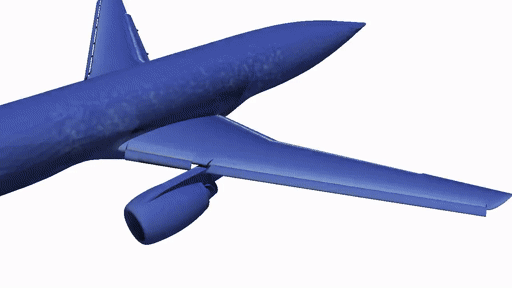#### Digital Math - reproducible research

We present the Digital Math framework as the foundation for modern science based on constructive digital mathematical computation. The computed result (coefficient vector, FEM function, plot, etc.) is a mathematical theorem, and the mathematical Open Source code, here in the FEniCS framework, and computation is the mathematical proof. We can also derive additional constructive proofs from the FEniCS and FEM formulation, such as stability.

Based on the Digital Math framework and the FEniCS realization, we present our Direct FEM Simulation (DFS) methodology together with predictions of the most advanced benchmarks available, unlocking the grand challenge of turbulence and aerodynamics.

Digital Math represents digitalization of mathematics, society, industry and society in the form of automated and easily understandable computation of mathematical models in the Open Source FEniCS framework with world-leading performance and recognized at the highest level of science and industry together with an effective pedagogical concept with combined abstract theory and mathematical interactive programming in a cloud-HPC web-interface.

#### Learning outcomes:

After this course the student should be able to:

1. Describe and demonstrate the technology needed for reproducible research in the Digital Math setting.

2. Create and publish their own reproducible research in the Digital Math setting.

#### Teachers

Johan Jansson (jjan@kth.se)

Arvind Kumar (arvkumar@kth.se)

#### Seminar

Presentation form of course page.

#### Learning activities

##### Automated Computational Modeling with FEniCS based on Finite Element Modeling - Fluid flow

Here's a screenshot of the Digital Math environment in Jupyter notebook: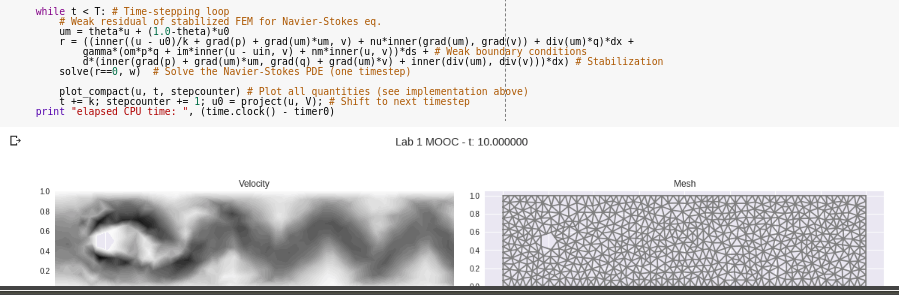##### Instructions

Do: Runtime->Run all

Observe the output, inspect the Digital Math, modify, re-run.

##### Examination

Create your own reproducible "Digital Math" publication as a Jupyter notebook, based on the examples and templates given here. For example, based on your interests, modify one of the examples, or create a new one from scratch. Publish it in Github, publically accessible and runnable.

Send your Jupyter notebook to Johan Jansson (jjan@kth.se), who will forward it to 1-2 other course participants to reproduce.

Try to reproduce (run and observe the result) the notebook sent to you. Send a few sentences to Johan Jansson (jjan@kth.se) describing if you could reproduce, or if not.

This examination is aligned with the learning outcomes, and is of the form "peer learning", which is considered one of the most effective pedagogical methods.

1. "Science needs to improve the transparency of research results, says report", PhysicsWorld, 2019
2. "Reproducibility and Replicability in Science", Lorena Barba, et. al., US National Academies of Sciences, Engineering and Medicine, 2019
3. Ioannidis, J. P. (2005). Why most published research findings are false. PLoS medicine, 2(8). (9000+ citations in 2020)
4. ”Kris i forskningsfrågan” , Hanne Kjöller, Fri Tanke, 2020.
5. "Reproducibility of scientific results in the EU", Directorate-General for Research and Innovation (European Commission), 2020
6. "Redefining research quality: Reproducibility and beyond", Ottersen, O. P., 2019
7. Events in the Macchiarini case, Karolinska Institutet, 2020
8. Digital Math, Johan Jansson, et. al., 2020
• #### The DigiMat Teacher Course Level 01

##### Learning goals

The DigiMat Teacher Course contains the following fundamental learning goals, which are the basis for all of Digital Math:

1. Number representation [Basic] in first binary form making representation and arithmetic algorithms easy to understand.
2. Arithmetic algorithms [Basic] constructed by repetition of the basic operation of +1 according the basic prototype of all computer programs of DigiMat in the form n = n + 1
3. Time-stepping [Basic-Pro] automatically solving all (ordinary) mathematical models in the form x = x + v*dt
4. Text programming [Basic-Pro] enabling the students to understand, modify and extend the algorithms and computer realizatons themselves.
##### Course Plan
1. Algorithms: binary addition and time stepping.
2. Accessible text programming. Concrete activities: time-stepping vibration in a guitar string, binary addition in the "falling stair case simulation".
3. Understanding and ability to yourself explain and demonstrate how programming of algoriths covers all of math - Digital Math.

Concrete example: time stepping implies solution of mathematical models (differential equations) such as harmonic oscillator, which can be interpreted as trigonometry.

4. Understanding and ability to yourself explain and demonstrate how time stepping covers all mathematical models (differential equations).

Concrete example: time stepping solves/simulates: harmonic oscillator, gravitation/planet system, mass-spring system (elastic bodies), wave propagation, fluid mechanics, etc.

5. Orientation of a large body of activities and material in DigiMat that build on 1-4 which you can adapt, build on yourself, etc. from your own interest and need to cover the entire course plan from pre-school to top academic level.

A large number of add-on courses can be made on this initial Teacher Course based on the material in 5.

##### Learning Activities
1. Watch the trailer video at the DigiMat main page!
2. Watch the introduction video to the right!
3. In Ada's World: Carry out the binary addition algorithm in the "falling stair case simulation".
4. In Ada's World: Carry out the timestepping algorithm in the "launching pinecone simulation".
5. In Ada's World: Carry out the text programming (creating your own dance) in the "dance simulation".
6. Text programming with time stepping. (Ada's World level 2 - DigiMat Pixel)

Watch the tutorial videos for "Text programming with time stepping" to the right. Now try yourself in Ada's World level 2 - DigiMat Pixel!

1. Draw two "digital objects", one will be red and one will be green.
2. In the "mathematical programming" box to the right, change the gravity force g to 0 ("g = 0"), and set the force on the green "digital object" to something you like, for example "fgreenx = 0.1". Play around with "fgreenx" and "fgreeny"! Try to set the values so the green object hits the red object!
7. Time-stepping vibration in a guitar string. (Ada's World level 2)

Play with the Digital Math code for the time-stepping. Choose different frequency/pitch values in the list, add and remove values, what happens?

Advanced: modify the "damping" to the guitar string by changing the term " - y*k" to the "y = y +"... line. What happens to the sound when you increase and decrease the damping?

##### Seminar 01.01 (flipped-classroom)

Tuesday October 13 16:00 CET Online together with the DigiMat team. Email jjan@kth.se if you want to participate!

For Seminar 01.01 we strongly encourage you to spend 20-30 minutes on Learning Activities 1-5. We will then have a high-level discussion in the Serminar, where you have the opportunity to learn from your peers by sharing your experiences, and to interact with the DigiMat team.

#### The DigiMat Teacher Course Level 02

##### Learning goals

The DigiMat Teacher Course contains the following fundamental learning goals, which are the basis for all of Digital Math:

1. Number representation [Basic] in first binary form making representation and arithmetic algorithms easy to understand.
2. Arithmetic algorithms [Basic] constructed by repetition of the basic operation of +1 according the basic prototype of all computer programs of DigiMat in the form n = n + 1
3. Time-stepping [Basic-Pro] automatically solving all (ordinary) mathematical models in the form x = x + v*dt
4. Text programing [Basic-Pro] enabling the students to understand, modify and extend the algorithms and computer realizatons themselves.
##### Course Plan

This is the course plan for DigiMat School which with good margin satisfies the new teaching plan 2018 in Sweden (with similar situations elsewhere globally) with programming as part of the math subject. The course plan has the same form for Middle School (Grundskola) and High School (Gymnasium), with differing breadth and depth.

Programming gives completely new possibilities to give the math subject meaning for all students since the student herself builds the math in interaction with the computing power of the computer in what can be described as building and playing of virtual interactive worlds and games.

Goal: To Describe-Simulate-Explore-Understand-Interact the Virtual World in the form of games and fantasy.

Language: Formal math notation + programming language such as JavaScript.

Content: Fantasy, Brain, Eyes, Fingers, Computer/Mobile device.

1. Text programming in languages such as JavaScript, Python. Fundamental algorithms and data structures: variable, list, array, function, repetition, conditional, logic.
2. Construction of natural numbers through iteration x = x + 1 med start x = 0
3. Computing with natural numbers: addition, subtraction, multiplication.
4. Text programming of computational algorithms in 2. Digital number representation starting with binary and then several bases.
5. Digital construction of rational numbers as solutions to the equation px = q with p and q natural numbers. Text programming of computational algorithms for rational numbers: addition, subtraction, multiplication and division.
6. Number representation of spatial position x: coordinate system, computer screen/pixels
7. Motion: change of position: x = x + v*dt (or dx = v*dt) with v velocity and dt time steo.
8. Change of motion: v = v + a*dt (or dv = a*dt) with a acceleration. Newton's second law: a = f/m where f is force and m mass.
9. Digital construction of elementary functions (polynomials, sin, cos, exp, log, etc.) through text programming of time stepping of a = f/m, v = v + a*dt, x = x + v*dt with simple f.
10. Properties of elementary functions through construction. Generalization to general f, for example by Newton's law of gravity.
11. Digital representation of and operation on geometric objects in 2D and 3D.
12. Digital representation of and operation on images and sounds, or other data.
13. Calculus as solution of dx = f(x)*dt
14. Linear algebra as generalization of 11.
15. Construction/text programming of interactive virtual worlds/games which build on 1-14 with for example the following components:
1. Dynamics of many bodies interacting by forces: friction, springs, gravity.
2. Interaction via buttons, knobs, touch or accelerometer/gyroscope.
3. Examples as Pong, Angry Birds, Flight Simulator, Pinball, Racing, Shooting, Pettson's Inventions, BikeRace, GeometryDash, Pool, Tennis, FlyBird, with and without use of physics engine.
##### Learning Activities

Follow the list of learning activities listed under DigiMat Basic and DigiMat School above!

##### Seminar 02.01 (flipped-classroom)

TBA Online together with the DigiMat team. Email jjan@kth.se if you want to participate!